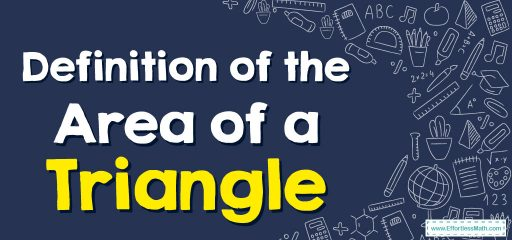# Area of a Triangle

The area of a triangle is the measure of the space enclosed within the triangle. To find the area of a triangle, you need to know the length of its base and its height.## A step-by-step guide to the definition of the area of a triangle

The area of a triangle is the amount of space that is enclosed within the three sides of the triangle.

To calculate the area of a triangle, you need to know the length of its base and its height.

The base of a triangle can be any of its sides, and the height is the perpendicular distance from the base to the opposite vertex.

The formula for finding the area of a triangle is:

Area = (base × height) / 2

To use this formula, you simply substitute the values of the base and height into the equation and then solve for the area. It’s important to use the same unit of measurement for both the base and height.

For instance, if the base of a triangle is 6 cm and the height is 4 cm, you can find the area using the formula:

Area = (6 cm × 4 cm) / 2 = 12 cm²

Therefore, the area of the triangle is 12 square centimeters.

### Definition of the Area of a Triangle – Example 1

Find the area of this triangle.

Solution:
Step 1: Multiply the base by the height. $$9×6=54$$
Step 2: Multiply $$\frac{1}{2}$$ by the result. $$\frac{1}{2}×54=27$$
So, the area of the triangle is $$27 ft^2$$.
Area$$=$$base$$×$$height$$×\frac{1}{2}→A=\frac{1}{2}(b×h)→A=\frac{1}{2}(9×6)=27$$

### Definition of the Area of a Triangle – Example 2

Find the area of this triangle.

Solution:
Step 1: Multiply the base by the height. $$14×8=112$$
Step 2: Multiply $$\frac{1}{2}$$ by the result. $$\frac{1}{2}×112=56$$
So, the area of the triangle is $$56 cm^2$$.
Area$$=$$base$$×$$height$$×\frac{1}{2}→A=\frac{1}{2}(b×h)→A=\frac{1}{2}(14×8)=56$$

### What people say about "Area of a Triangle - Effortless Math: We Help Students Learn to LOVE Mathematics"?

No one replied yet.

X
30% OFF

Limited time only!

Save Over 30%

SAVE $5 It was$16.99 now it is \$11.99